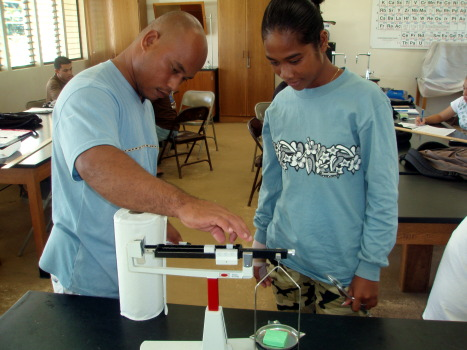MS 150 Statistics fall 2008 test one • Name:

Density
g/cm³
1.79
1.69
1.43
0.98
0.89
0.93
0.70
0.54
1.57
1.47
1.64
1.47
1.36
1.44
1.45
0.98
0.89
0.93
0.70
0.54

On 21 August 2008 students in SC 130 Physical Science measured the volume and mass of rectangular slabs of soap. This data was used to calculate the density of the soap slabs. The density data is reported in the table.1. __________ What is the level of measurement?
2. __________ Find the sample size n.
3. __________ Find the minimum.
4. __________ Find the maximum.
5. __________ Find the range.
6. __________ Find the midrange.
7. __________ Find the mode.
8. __________ Find the median.
9. __________ Find the sample mean x.
10. __________ Find the sample standard deviation sx.
11. __________ Find the sample coefficient of variation CV.
12. __________ If this data is to be divided into five classes, what is the width of a single class?
13. Determine the frequency and calculate the relative frequency using five classes. Record your results in the table provided.
Bin upper limitsFrequency FRelative Frequency
Sums:
1. Sketch a relative frequency histogram of the data, labeling your horizontal axis and vertical axis as appropriate.
2. __________ What is the shape of the histogram?
3. __________ Based on the histogram, how many different kinds of soap were measured by the SC 130 physical science class?
4. z = __________ Some students reported densities for their soap slabs as high as 2.48 g/cm³. Use the formula z = (x - x)/sx to find the z-score for a slab that had a density of 2.48 g/cm³.
5. _________________ Is a density of 2.48 g/cm³ an ordinary value or an unusual (extraordinary) value based on the sample data above?
6. _________________ Based on the above analysis, would we conclude that 2.48 g/cm³ is an accurate measurement or would we conclude that 2.48 g/cm³ is the result of measuring errors?